# Datathon Telenor Solution – Analysis Of Mobile Data Connectivity Delays

Problem statement :This data set is regarding time series analysis on failure rate of ravens sending the messages from king’s landing to the north . This case study is an analogy on Telenor telecommunications and Game of Thrones . Due to the obstacles that caused the failure rate , various techniques and schemes are employed in the planning, design and optimization of raven networks to combat these propagation effects.

We have used R-studio for Exploratory Data Analysis.
As per the tasks given to us , we concluded that
1.Brass Raven Birdy has been delayed for the most number of times , followed by Brown raven ruby and Yellow raven Rio,
while Metallic Sunburst Raven Polly has been delayed for the least number of times , followed by Green Sheen raven Azul and Less combative raven zazu.
2. The family with most fails is Targerian , while with least fails is Lannister
3. The family Member with most fails is Petyr Baelish and with least fails is Euron .
We have done further analysis on predicting the fails for the next four days using TIME SERIES ANALYSIS

2

datathon

# Analysis Of Mobile Data Connectivity Delays

##### Names – Aashi Agarwal, Ananya Jena , Devesh Tripathi , Prasad shripathi

The Telenor Case – What do Game of Thrones and Telecoms Have in Common?

• Team Toolset : R studio , Ms-Excel

DATA SCIENCE LIFECYCLE :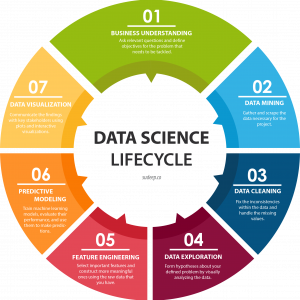Sending ravens is one of the most fundamental parameters in mobile communications engineering. For land-based mobile communications, the received raven variation is primarily the result of multipath fading caused by obstacles such as buildings (or clutter) or terrain irregularities; the distance between link end points; predatory animals, and interference among multiple transmissions, for example wars. This inevitable raven variation is the cause of communication dropping, one of the most significant quality of service measure in operative communication. For this reason, various techniques and schemes are employed in the planning, design and optimization of raven networks to combat these propagation effects. This normally covers the network physical configuration which include all aspects of network infrastructure deployment such as locations of base nests; additional food; sometimes guards, etc. A typical example of these schemes and techniques is the use of models for flight prediction based on measured data.

Based on one month data with flight fails, the we have to  make time-series analysis and predict the future amount of fails.

To analyse the data Set , we are required to perform these tasks through Exploratory Data Analysis :

-Top 10 ravens with fails

-Top 10 ravens without fails

-The family with most fails

-The family with least fails

-The family member with most fails

-The family member with least fails

## Data Understanding ::

We are working on the mobile data fails dataset.

As we analyse the data,

• Every row has fifteen minute basis communication
• It contains information about which raven made the communication and by which network (2G/3G/4G).
• Also, who initiated the communication – family and member name
• Different types of fails – sum of delays.

–>The given dataset contains 22401305 rows and 16 columns in CSV file format , the following columns represent :

1. DateTime : At what date and time , the raven made the communication.
2. Raven_name : Name of the Raven which made the communication
3. Family_name : It refers to the person’s Family Name who has initiated the communication.
4. Member_name : It refers to the person’s name who has initiated the communication .
5. Network : It contains information about the raven that made the communication through which network (2G/3G/4G).

THE REST ALL COLUMNS (i.e from column 6 to column 16) ARE REPRESENTING DIFFERENT TYPES OF DELAYS. THERE ARE 11 SUCH COLUMNS THAT ARE

1. FIRST_GET_RESPONSE_SUCCESS_D
2. PAGE_BROWSING_DELAY
3. TCP_SETUP_TOTAL_DELAY
5. FIRST_DNS_RESPONSE_SUCCESS_D
6. DNS_RESPONSE_SUCCESS_DELAY
7. FIRST_TCP_RESPONSE_SUCCESS_D
8. PAGE_SR_DELAYS14. SYN_SYN_DELAY
9. TCP_CONNECT_DELAY
10. PAGE_BROWSING_DELAYS

In above columns, ‘0’ signifies  success of the message being passed without any delay .

## Reading the Data and Preprocessing:

### Task 1: Top 10 ravens with fails

#rcode ::

total_delay_with_fails = data%>%filter(FIRST_GET_RESPONSE_SUCCESS_D !=0 | PAGE_BROWSING_DELAY !=0 |
FIRST_DNS_RESPONSE_SUCCESS_D !=0 | DNS_RESPONSE_SUCCESS_DELAY !=0 |
FIRST_TCP_RESPONSE_SUCCESS_D !=0 | PAGE_SR_DELAYS !=0 |
SYN_SYN_DELAY !=0 | TCP_CONNECT_DELAY !=0 | PAGE_BROWSING_DELAYS !=0)%>%
group_by(RAVEN_NAME)%>%
summarise(count = n())%>%
arrange(-count)%>%

View(total_delay_with_fails)

### Task 2 :  Top 10 ravens without fails

#rcode ::

total_delay_without_fails = data%>%filter(FIRST_GET_RESPONSE_SUCCESS_D ==0 & PAGE_BROWSING_DELAY ==0 &
FIRST_DNS_RESPONSE_SUCCESS_D ==0 & DNS_RESPONSE_SUCCESS_DELAY ==0 &
FIRST_TCP_RESPONSE_SUCCESS_D ==0 & PAGE_SR_DELAYS ==0 &
SYN_SYN_DELAY ==0 & TCP_CONNECT_DELAY ==0 & PAGE_BROWSING_DELAYS ==0)%>%
group_by(RAVEN_NAME)%>%
summarise(count = n())%>%
arrange(-count)

View(total_delay_without_fails)
View(top_10_without_fails)

### Task 3 : The family with most fails

#rcode ::

family_with_fails = data%>%filter(FIRST_GET_RESPONSE_SUCCESS_D !=0 & PAGE_BROWSING_DELAY !=0 &
FIRST_DNS_RESPONSE_SUCCESS_D !=0 & DNS_RESPONSE_SUCCESS_DELAY !=0 &
FIRST_TCP_RESPONSE_SUCCESS_D !=0 & PAGE_SR_DELAYS !=0 &
SYN_SYN_DELAY !=0 & TCP_CONNECT_DELAY !=0 & PAGE_BROWSING_DELAYS !=0)%>%
group_by(FAMILY_NAME)%>%
summarise(count = n())%>%
arrange(-count)
View(family_with_fails)
View(family_with_most_fails)

### Task 4:: The family with least fails

#rcode ::

family_with_least_fails = family_with_fails%>%tail(1)
View(family_with_least_fails)

### Task 5 :: The family member with most fails

#rcode ::
family_member_with_fails = data%>%filter(FIRST_GET_RESPONSE_SUCCESS_D !=0 & PAGE_BROWSING_DELAY !=0 &
FIRST_DNS_RESPONSE_SUCCESS_D !=0 & DNS_RESPONSE_SUCCESS_DELAY !=0 &
FIRST_TCP_RESPONSE_SUCCESS_D !=0 & PAGE_SR_DELAYS !=0 &
SYN_SYN_DELAY !=0 & TCP_CONNECT_DELAY !=0 & PAGE_BROWSING_DELAYS !=0)%>%
group_by(MEMBER_NAME)%>%
summarise(count = n())%>%
arrange(-count)
View(family_member_with_fails)
View(family_member_with_most_fails)

### Task 6 ::The family member with least fails

#rcode :

family_member_with_least_fails = family_member_with_fails%>%tail(1)
View(family_member_with_least_fails)

## EDA – grouping and plotting :

For task1 :Top 10 ravens with fails

ggplot(top_10_delay_with_fails, aes(x=RAVEN_NAME, y=count, fill=count))+
geom_segment(data = top_10_delay_with_fails, aes(x=RAVEN_NAME, xend=RAVEN_NAME, y=0, yend=count), linetype=’solid’, color=’orchid4′, size=5) +
geom_label(aes(label=count), color=’white’, size=4, vjust=-0.9, label.size = 0, fontface=’bold’ )+
coord_flip()+ theme_gray()+
theme(axis.text.y = element_text(face=’bold’))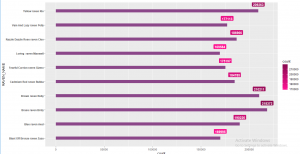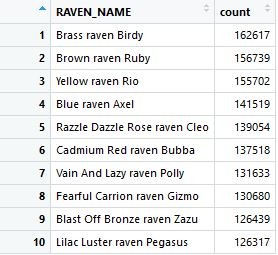Conclusion : These are the top 10 ravens that were failed(delayed). Brass Raven Birdy has been failed(or delayed) for the most number of times i.e. 162617 , followed by Brown raven ruby i.e. 156739 , then Yellow raven Rio i.e. 155702, Blue raven Axel : 141519 , Razzle Dazzle Rose raven Cleo : 139054 , Cadmium Red raven Bubba : 137518 , Vain And Lazy raven Polly :131633 , Fearful Carrion raven Gizmo :130680 , Blast Off Bronze raven Zazu : 126439 , Lilac Luster raven Pegasus : 126317 .

For task 2 : Top 10 ravens without fail

ggplot(top_10_without_fails, aes(x=RAVEN_NAME, y=count, fill=count))+
geom_segment(data = top_10_without_fails, aes(x=RAVEN_NAME, xend=RAVEN_NAME, y=0, yend=count), linetype=’solid’, color=’orchid4′, size=5) +
geom_label(aes(label=count), color=’white’, size=4, vjust=-0.9, label.size = 0, fontface=’bold’ )+
coord_flip()+ theme_gray()+
theme(axis.text.y = element_text(face=’bold’))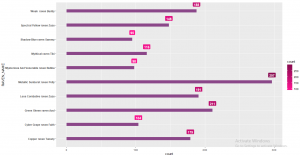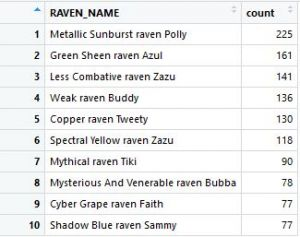Conclusion : These are the top 10 ravens that were not  failed(delayed) . Metallic Sunburst raven Polly : 225 , Green Sheen raven Azul : 161 , Less Combative raven Zazu : 141 ,Weak raven Buddy : 136 , Copper raven Tweety :130 , Spectral Yellow raven Zazu : 118 , Mythical raven Tiki : 90 , Mysterious And Venerable raven Bubba : 78,  Cyber Grape raven Faith : 77 , Shadow Blue raven Sammy : 77

For task 3 & 4:The family with most fails and with least fails

ggplot(family_with_fails, aes(x= reorder(FAMILY_NAME,-count), y = count))+
geom_bar(stat = ‘Identity’, width = 0.5,aes(fill = FAMILY_NAME))+theme_bw()+
geom_text(aes(label=count), vjust=-0.3, size=3.5)+
labs(title = “FAMILY WITH MOST AND LEAST FAILS”, subset = “delays”, x = “family name”, y = “total fails”)+
theme(legend.position = “right”)+theme(legend.key.width = unit(.5,”cm”),legend.key.height = unit(.5,”cm”))+
theme(legend.title = element_blank())+
theme(axis.text.x = element_blank(),axis.ticks.x = element_blank(),axis.title.x = element_blank())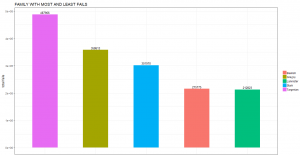Conclusion : This is the family having the most number of fails i.e Targerian with 363100 fails and The family having the least number of fails i.e Lannister with 158227 fails .

For task 5 : The family member with most fails

ggplot(family_member_with_most_fails, aes(x= reorder(MEMBER_NAME,-count), y = count))+
geom_bar(stat = ‘Identity’, width = 0.5,aes(fill = MEMBER_NAME))+theme_bw()+
geom_text(aes(label=count), vjust=-0.3, size=3.5)+
labs(title = “FAMILY MEMBER WITH MOST FAILS”, subset = “delays”, x = “member name”, y = “total fails”)+
theme(legend.position = “right”)+theme(legend.key.width = unit(.5,”cm”),legend.key.height = unit(.5,”cm”))+
theme(legend.title = element_blank())+
theme(axis.text.x = element_blank(),axis.ticks.x = element_blank(),axis.title.x = element_blank())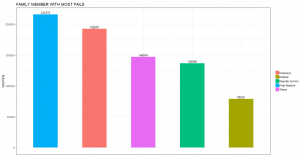Conclusion : The family member with most number of fails is  Petyr Baelish with 160210

For task 6: The family member with least fails

ggplot(family_member_with_least_fails, aes(x= reorder(MEMBER_NAME,-count), y = count))+
geom_bar(stat = ‘Identity’, width = 0.5,aes(fill = MEMBER_NAME))+theme_bw()+
geom_text(aes(label=count), vjust=-0.3, size=3.5)+
labs(title = “FAMILY MEMBER WITH LEAST FAILS”, subset = “delays”, x = “member name”, y = “total fails”)+
theme(legend.position = “right”)+theme(legend.key.width = unit(.5,”cm”),legend.key.height = unit(.5,”cm”))+
theme(legend.title = element_blank())+
theme(axis.text.x = element_blank(),axis.ticks.x = element_blank(),axis.title.x = element_blank())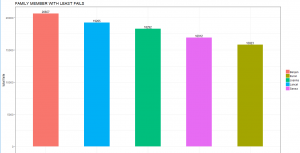Conclusion : The family member with least  number of fails is  Euron with 11759.

## Time Series Forecasting

### Objectives :

• Plotting, analyzing, and preparation of series for modeling
• Testing for stationarity and applying appropriate transformations
• Decide on the order of an ARIMA model
• Forecast the series
##### ARIMA model::

Based on one month data with browsing fails, We have to use time-series analysis and to predict the future amount of fails.

The following code in done using R Studio ::

total_date = data%>%filter(FIRST_GET_RESPONSE_SUCCESS_D !=0 & PAGE_BROWSING_DELAY !=0 &
FIRST_DNS_RESPONSE_SUCCESS_D !=0 & DNS_RESPONSE_SUCCESS_DELAY !=0 &
FIRST_TCP_RESPONSE_SUCCESS_D !=0 & PAGE_SR_DELAYS !=0 &
SYN_SYN_DELAY !=0 & TCP_CONNECT_DELAY !=0)%>%
group_by(DATETIME)%>%
summarise(count = n())
View(total_date)

##DateTime series
##Using ts function we are assigning date time series on count i.e sating that failure depends on date-time
##column.assigning start date and end date to run the model for that period

date_ts =ts(total_date\$count, start =c(2018, 7, 6), end = c(2018, 8, 5), frequency = 15)

##Preparing the difference variable
d_total_sum = diff(total_date\$count)

## estimating AR model
fit_diff_ar = arima(d_total_sum, order = c(1, 0, 2))
d_total_sum = diff(d_total_sum, 96)
acf(d_total_sum)
pacf(d_total_sum)
fit_diff_ar = arima(d_total_sum, order = c(1, 0, 2))
fit_diff_ar
#(p,d,q)

#(1,0,1)
#(1,0,2)
#(2,0,1)
#(2,0,2)
AIC(arima(d_total_sum,c(1,0,1)))
AIC(arima(d_total_sum,c(1,0,2)))
AIC(arima(d_total_sum,c(2,0,1)))
AIC(arima(d_total_sum,c(2,0,2)))

d = ndiffs(d_total_sum)
auto.arima(d_total_sum)
## forcasting
fit_diff_arf = forecast(fit_diff_ar, h = 384)
plot(forecast(fit_diff_ar, h = 384))
model = nnetar(d_total_sum)
nnetar(d_total_sum)
temp = forecast(object = model,h = 384)
plot(temp, 384)

## Forecasting from ARIMA daywise

data2 = data%>%filter(FIRST_GET_RESPONSE_SUCCESS_D !=0 & PAGE_BROWSING_DELAY !=0 &
FIRST_DNS_RESPONSE_SUCCESS_D !=0 & DNS_RESPONSE_SUCCESS_DELAY !=0 &
FIRST_TCP_RESPONSE_SUCCESS_D !=0 & PAGE_SR_DELAYS !=0 &
SYN_SYN_DELAY !=0 & TCP_CONNECT_DELAY !=0)%>%
group_by(DATETIME, MEMBER_NAME) %>%
summarise(total = n())

extract = as.Date(data2\$DATETIME, “%Y-%m-%d %H:%M”)
data2\$Date = format(extract, “%Y-%m-%d”)
View(data2)

data3 = data2%>%group_by(Date)%>%summarise(total.sum = sum(total))
sum(data3\$total.sum)
View(data3)

date_ts =ts(data3\$total.sum, start =c(2018, 7, 6), end = c(2018, 8, 5), frequency = 1)
d_total_sum = diff(data3\$total.sum, 1)

fit_diff_ar = arima(d_total_sum, order = c(1, 0, 2))

auto.arima(d_total_sum)
fit_diff_ar
## forcasting
fit_diff_arf = forecast(fit_diff_ar, h = 4)
plot(forecast(fit_diff_ar, h = 4))

PLOTTING: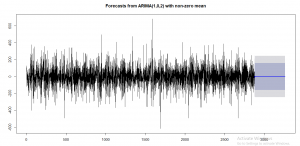####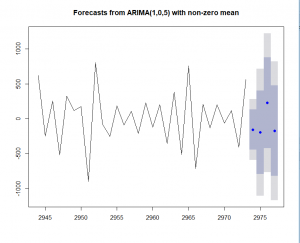Here , x axis represents Date- Time ,

y axis represents the number of fails